FEATool Multiphysics  v1.15 Finite Element Analysis Toolbox
Non-Newtonian Flow in an Extrusion Die

This example models flow of a polymer through an extrusion die with two outlets. This type of flow can for example be found in manufacturing processes of plastic parts. The polymer is assumed to be non-Newtonian, and can be modeled with a shear thinning Bird-Carreau viscosity model. The die is also assumed to be rotationally symmetric, allowing a reduction of the model geometry to an axisymmetric cross section.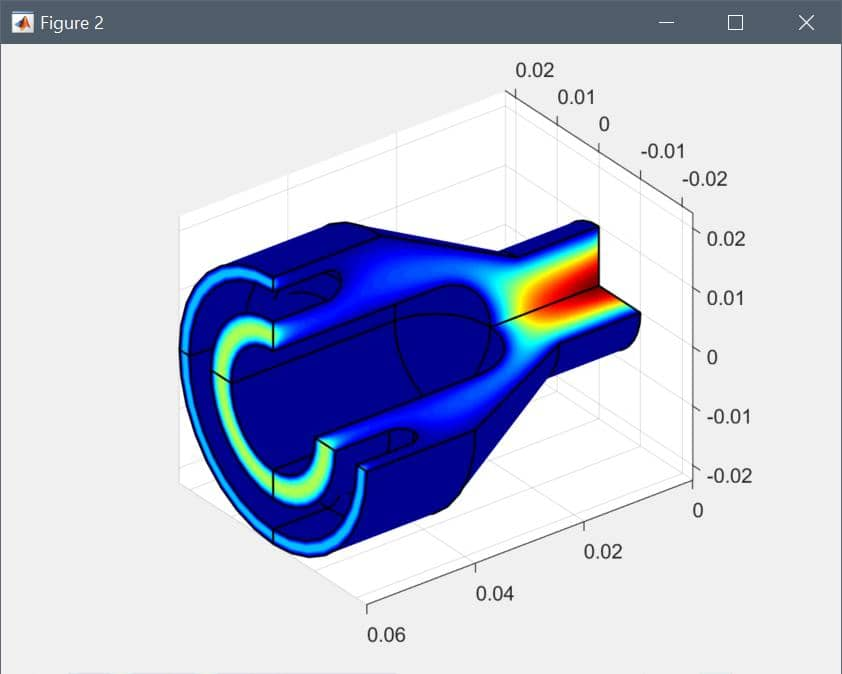Simulation of Non-Newtonian Flow in an Extrusion Die

# Tutorial

This model is available as an automated tutorial by selecting Model Examples and Tutorials... > Fluid Dynamics > Non-Newtonian Flow in an Extrusion Die from the File menu, viewed as a video tutorial, or alternatively, follow the step-by-step instructions below.

1. To start a new model click the New Model toolbar button, or select New Model... from the File menu.
2. Select the Axisymmetry radio button.
3. Select the Non-Newtonian Flow physics mode from the Select Physics drop-down menu.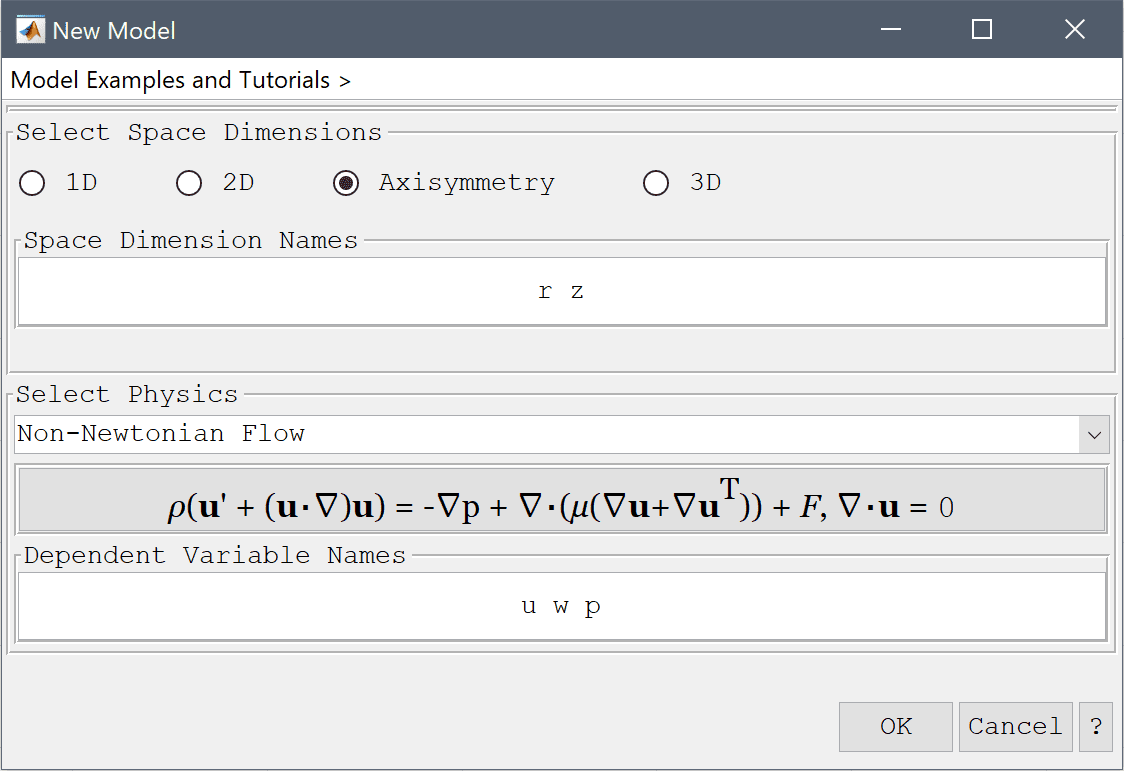4. Press OK to finish the physics mode selection.
5. The cross section of the geometry can be constructed by using the polygon tool, and subtracting two cylinders.
6. Select Polygon from the Geometry menu.

Enter the following data into the Point coordinates table.

r z
1 0 0
2 0.01 0
3 0.01 0.015
4 0.0225 0.04
5 0.0225 0.06
6 0.02 0.06
7 0.02 0.05
8 0.015 0.05
9 0.015 0.06
10 0.01 0.06
11 0.01 0.03
12 0 0.02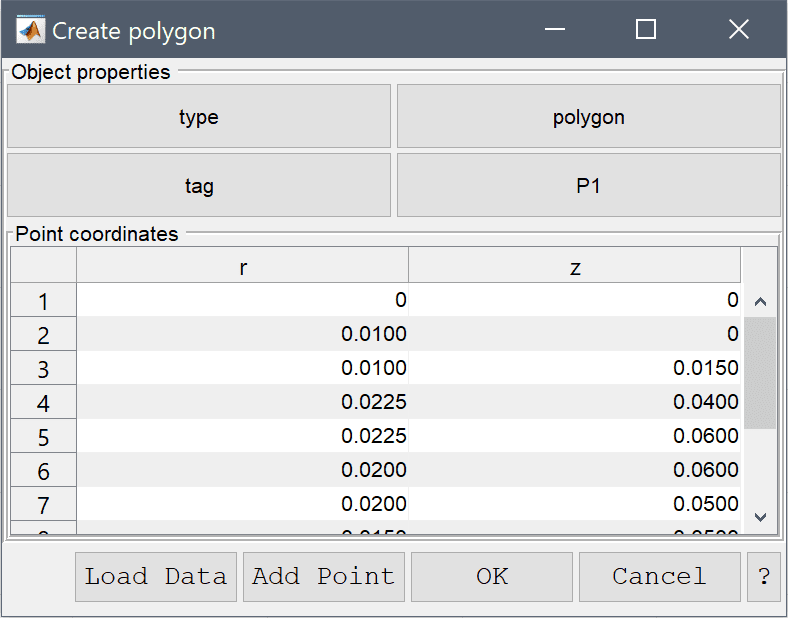1. Press OK to finish and close the dialog box.
2. Select Circle from the Geometry menu.
3. Enter 0 0.03 into the center edit field.
4. Enter 0.01 into the radius edit field.
5. Press OK to finish and close the dialog box.
6. Select Circle from the Geometry menu.
7. Enter 0.0175 0.05 into the center edit field.
8. Enter 0.0025 into the radius edit field.
9. Press OK to finish and close the dialog box.
10. Select P1, C1, and C2 in the geometry object Selection list box.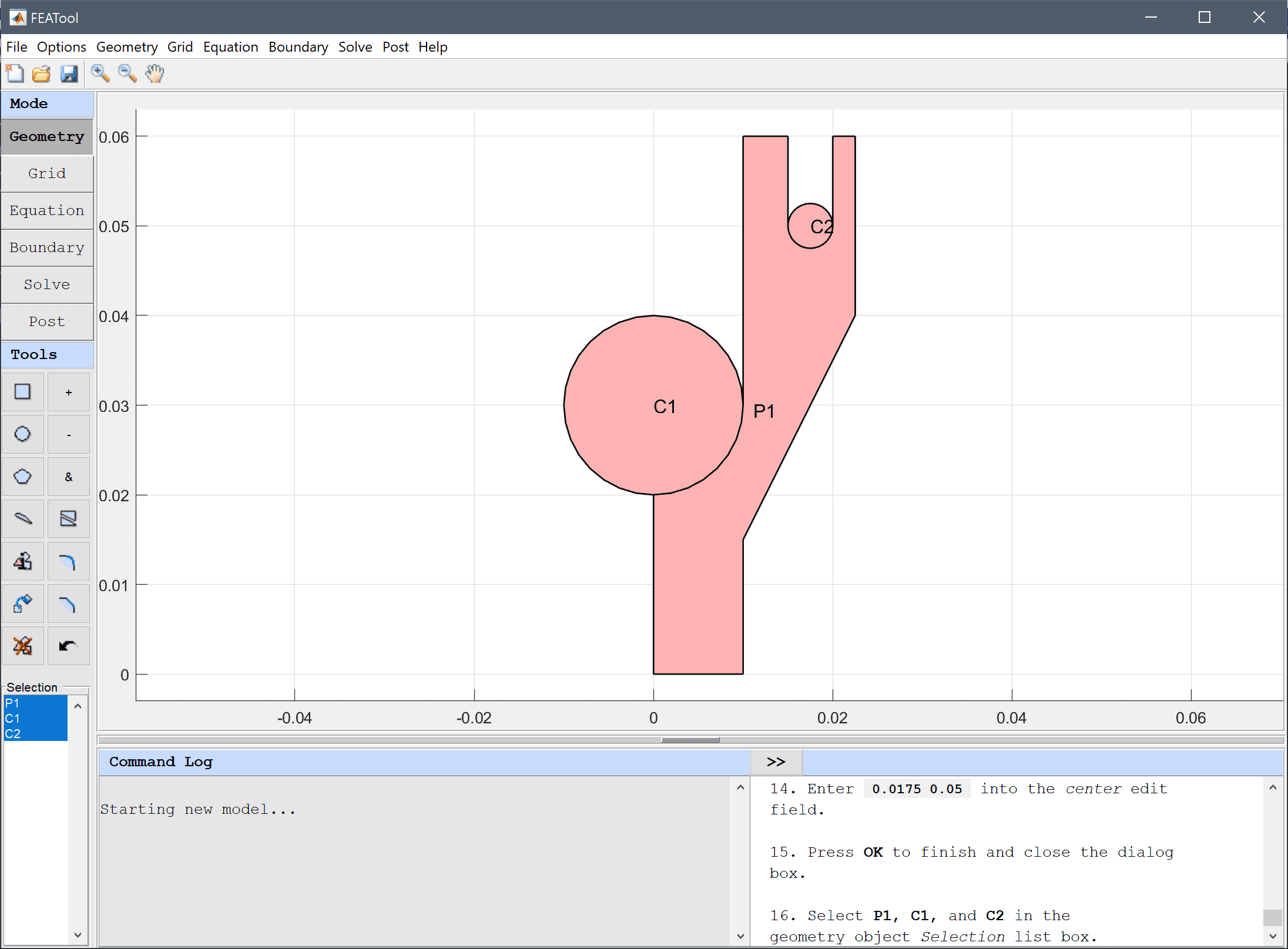11. Press the - / Subtract geometry objects Toolbar button.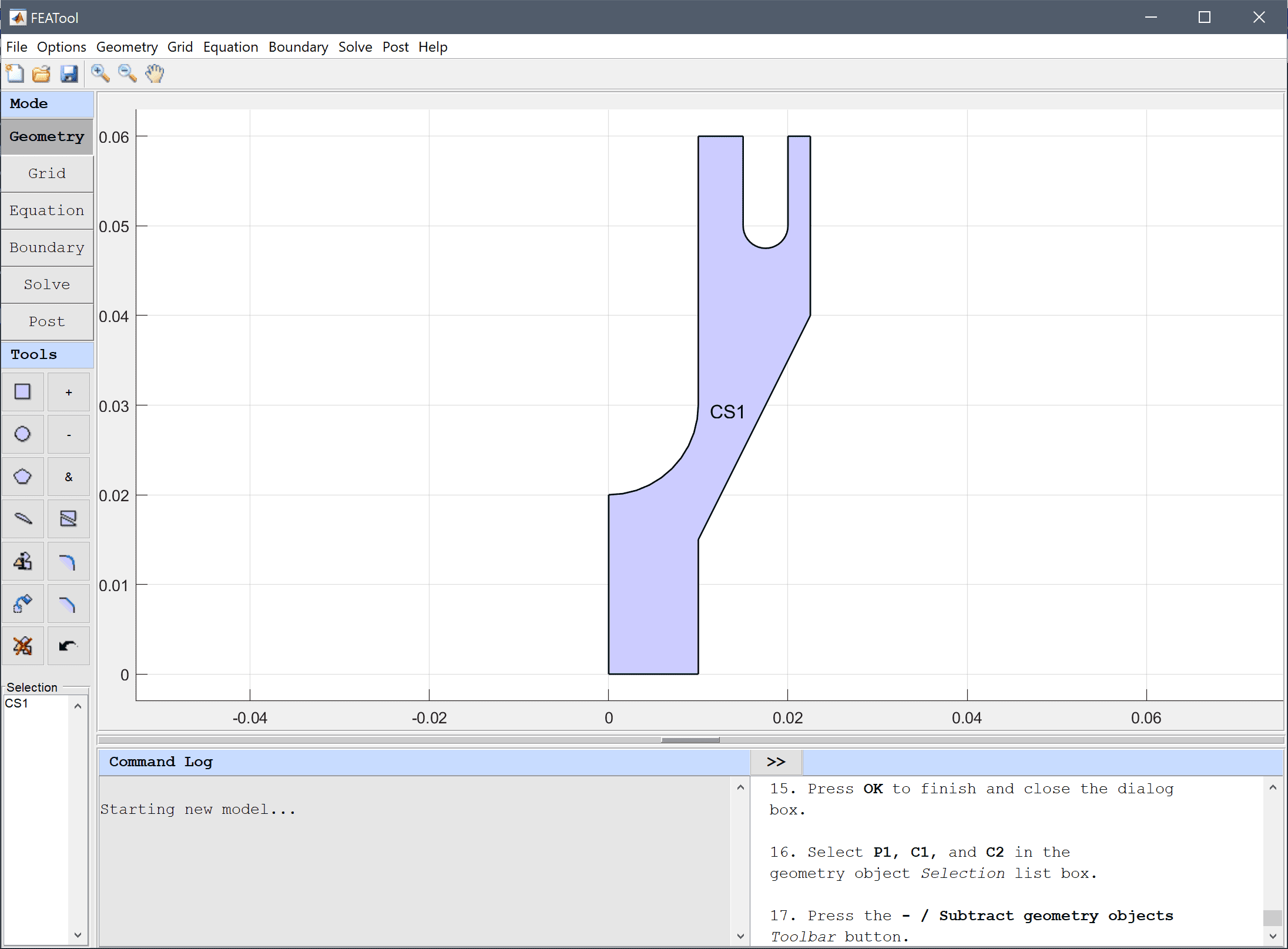12. Switch to Grid mode by clicking on the corresponding Mode Toolbar button.
13. The default grid may be too coarse to ensure an accurate solution. Decrease the grid size to 8e-4 to generate a finer grid that can resolve the curved boundaries better.
14. Press the Generate button to call the automatic grid generation algorithm.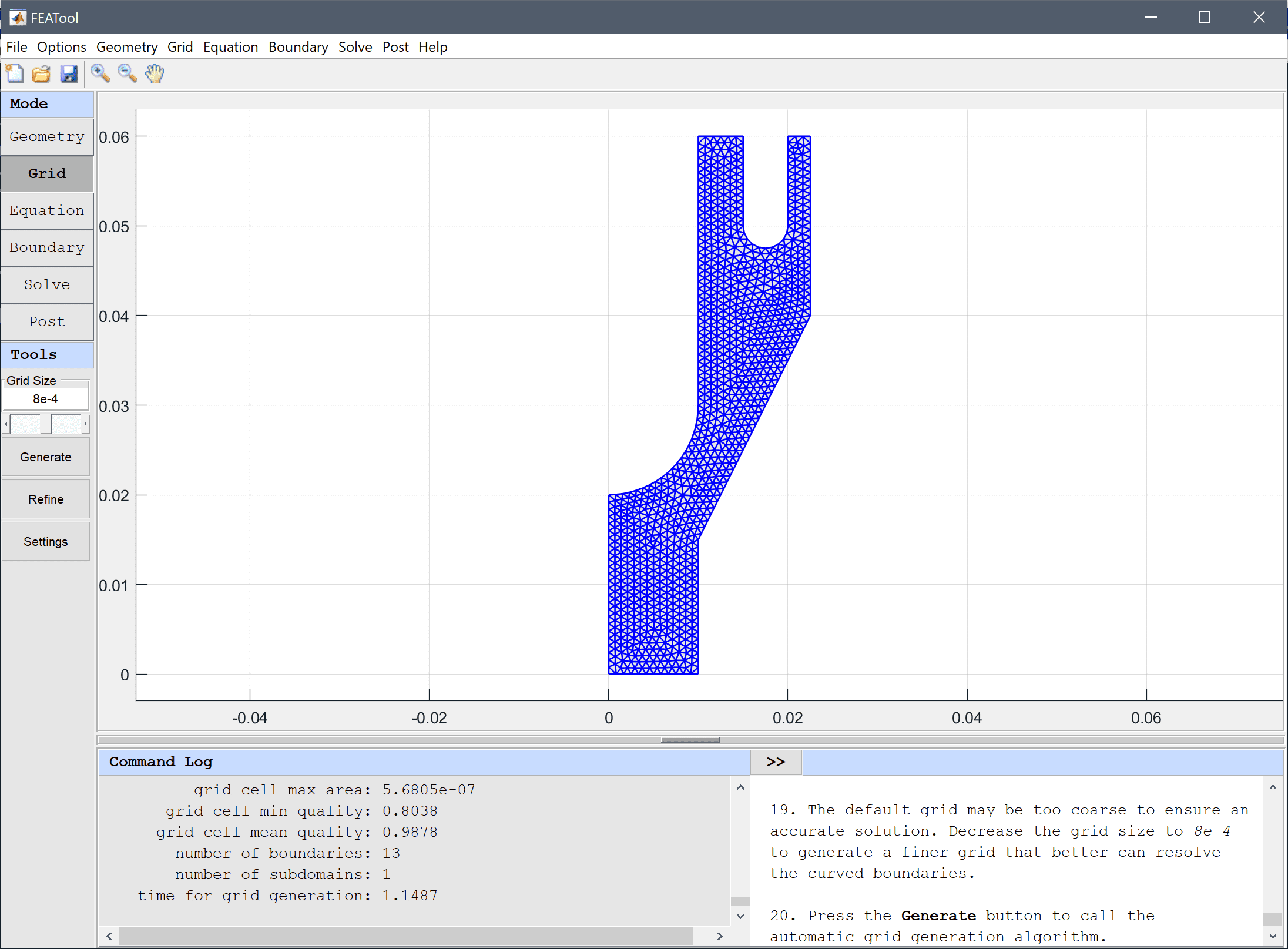15. Switch to Equation mode by clicking on the corresponding Mode Toolbar button.

Equation and material coefficients can be specified in Equation/Subdomain mode.

Note that FEATool works with any unit system, and it is up to the user to use consistent units for geometry dimensions, material, equation, and boundary coefficients.

1. Enter 510 into the Density edit field.

The Bird-Carreau viscosity model is used here, with a zero shear rate viscosity of 130 Pa s, relaxation time of K = 0.015 1/s, and power index n = 0.43 (indicating a shear thinning pseudo plastic flow regime).

1. Select Bird-Carreau from the Viscosity model drop-down menu.

Note that the expression in the edit field for the viscosity now has been updated with the chosen viscosity model.

1. Enter 130 into the Reference viscosity edit field.
2. Enter 0 into the Viscosity at infinite shear rate edit field.
3. Enter 0.015 into the Model parameter edit field.
4. Enter 0.43 into the Power law index edit field.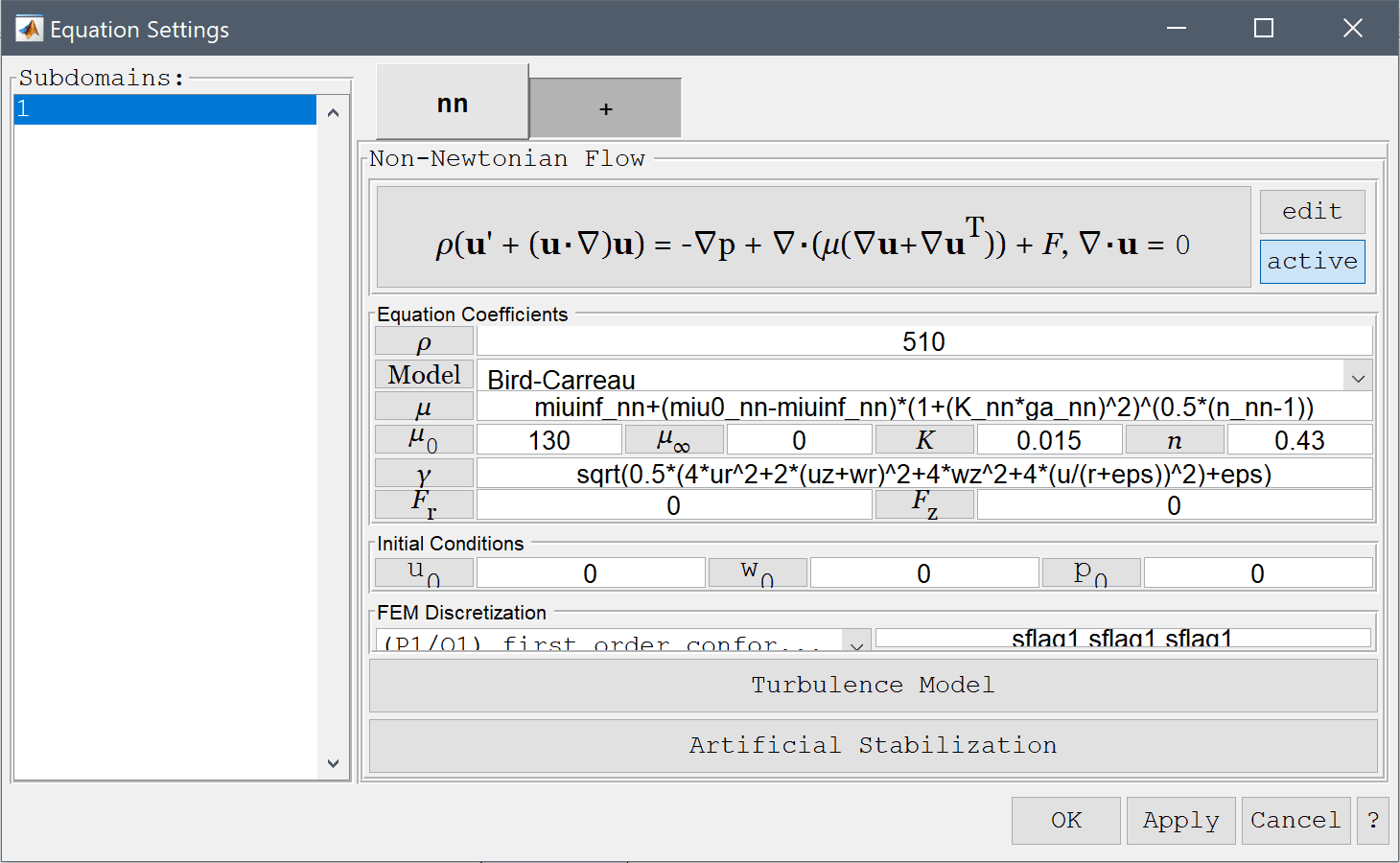5. Press OK to finish the equation and subdomain settings specification.
6. Switch to Boundary mode by clicking on the corresponding Mode Toolbar button.

A differential pressure of 2e4 Pa is applied between the inlet and the two outlets.

1. Select 1 in the Boundaries list box.
2. Select Outflow/pressure from the drop-down menu.
3. Enter 2e4 into the Pressure edit field.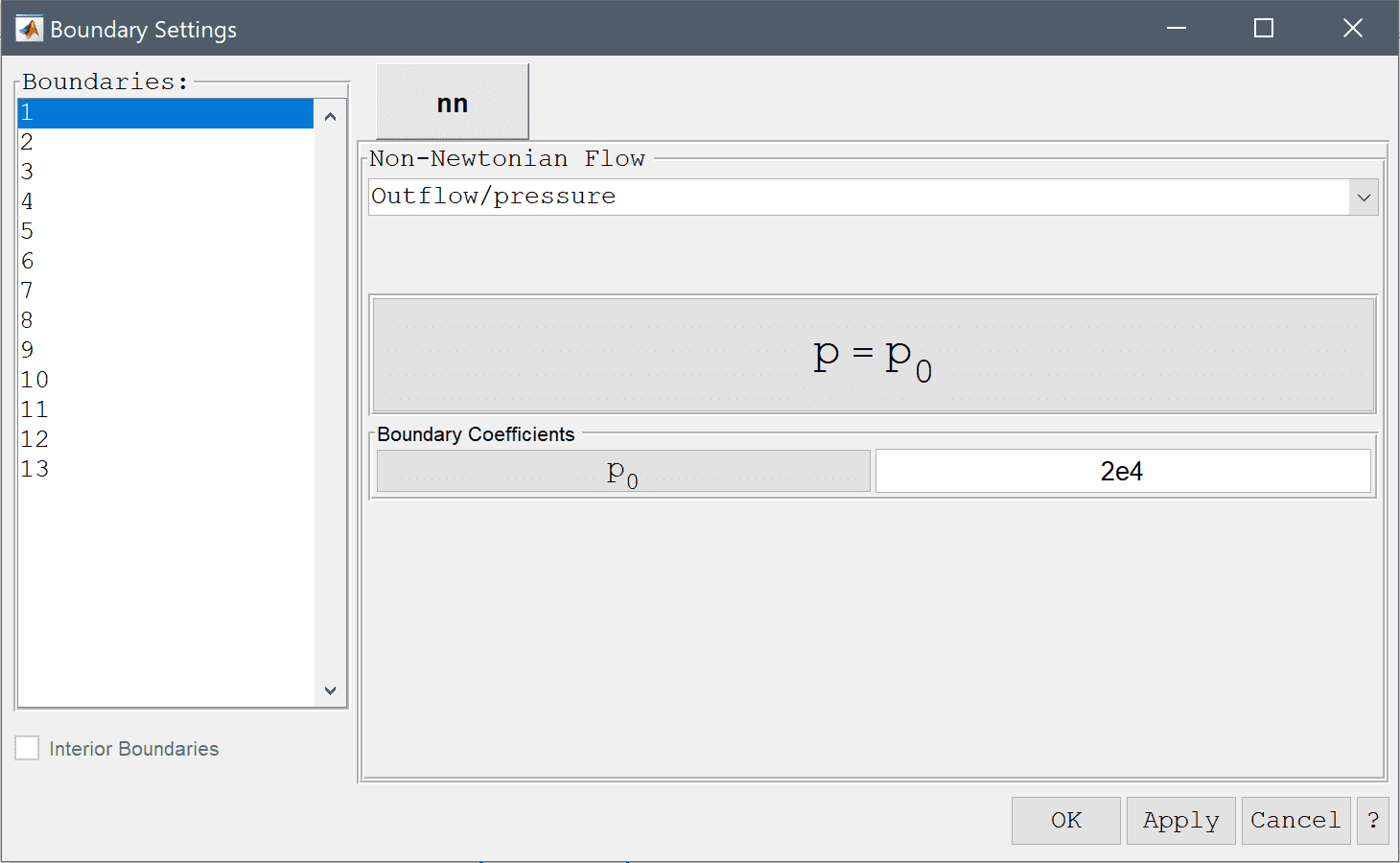4. Select 5 and 10 in the Boundaries list box.
5. Select Neutral outflow/stress boundary from the drop-down menu.

Boundaries along the symmetry axis _(r = 0)_ must be set to the Symmetry and slip condition.

1. Select 13 in the Boundaries list box.
2. Select Symmetry/slip from the drop-down menu.
3. Press OK to finish the boundary condition specification.
4. Now that the problem is fully specified, press the Solve Mode Toolbar button to switch to solve mode. Then press the = Tool button to call the solver with the default solver settings.
5. After the problem has been solved FEATool will automatically switch to postprocessing mode, and display the computed velocity field. To change the plot, open the postprocessing settings dialog box by clicking on the Plot Options Toolbar button.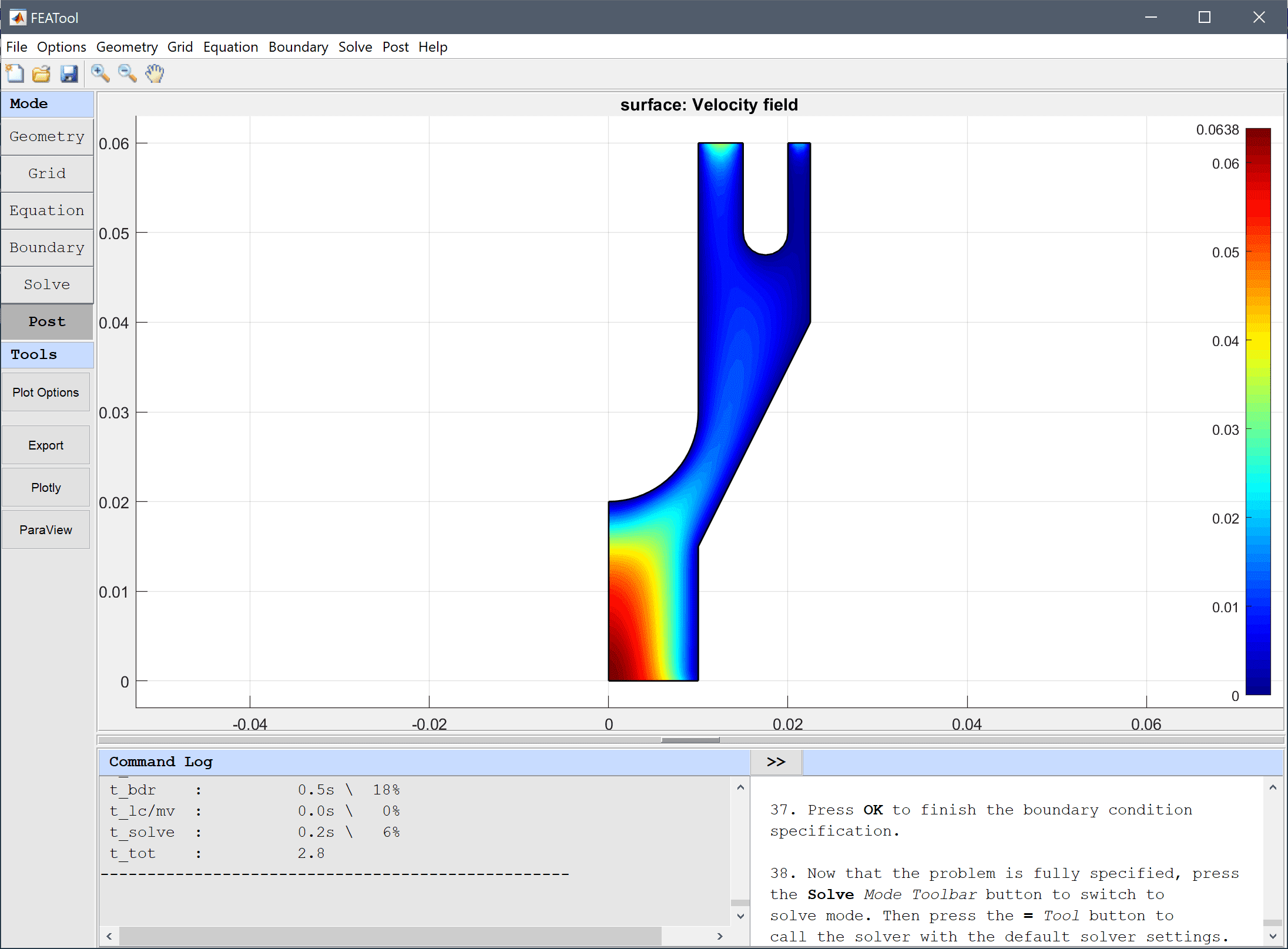Visualize the effective viscosity and the flow field as arrows.

1. Select Effective viscosity from the Predefined surface plot expressions drop-down menu.
2. Select the Enable/disable arrow plot check box.
3. Enter 20 into the Arrow spacing in the r-direction edit field.
4. Enter 50 into the Arrow spacing in the z-direction edit field.
5. Select white from the Select arrow color drop-down menu.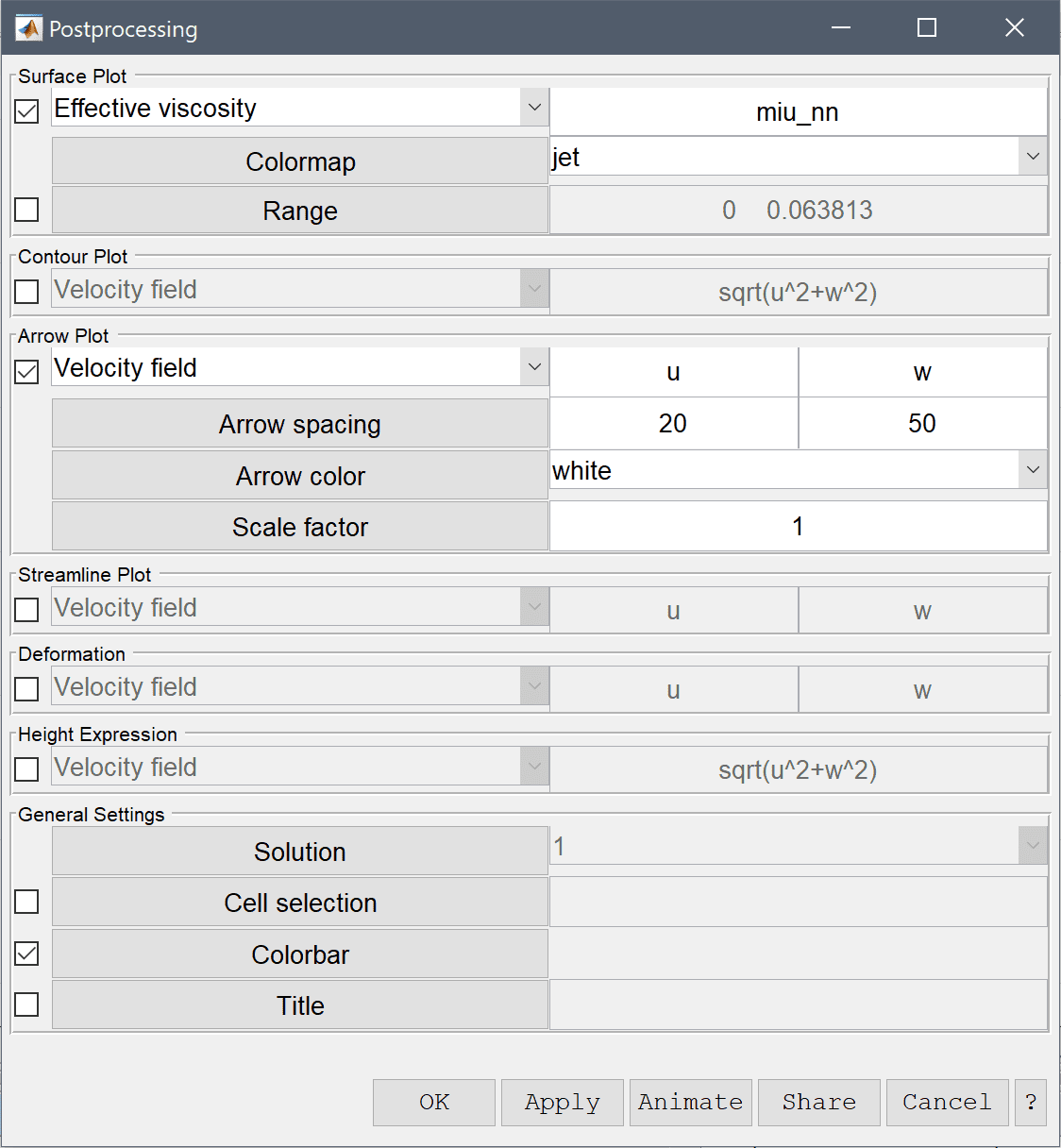6. Press OK to plot and visualize the selected postprocessing options.

The effective viscosity is decreased in regions where the velocity field exhibit gradients as is expected for shear thinning fluids.

We can also use the line plot functionality to plot and see the difference in the flow field at the outlets.

1. Select Point/Line Evaluation... from the Post menu.
2. Select Velocity field from the Evaluation Expression drop-down menu.
3. Enter an expression for a vector from 0.01 to 0.025 in steps of 1e-4, 0.01:1e-4:0.025, in the edit field for Evaluation coordinates in the r-direction.
4. Similarly, enter an expression with a corresponding amount of points at z = 0.06 , 0.06*ones(1,150), in the edit field for Evaluation coordinates in the z-direction.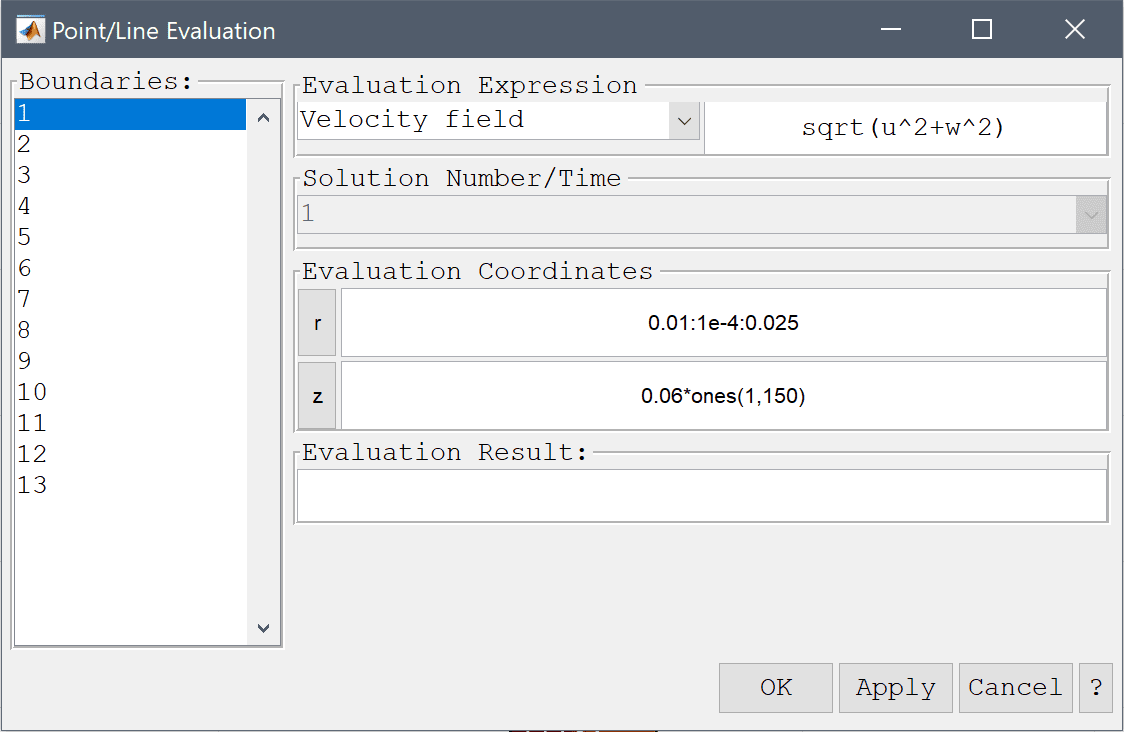5. Press OK to plot the curve and close the dialog box.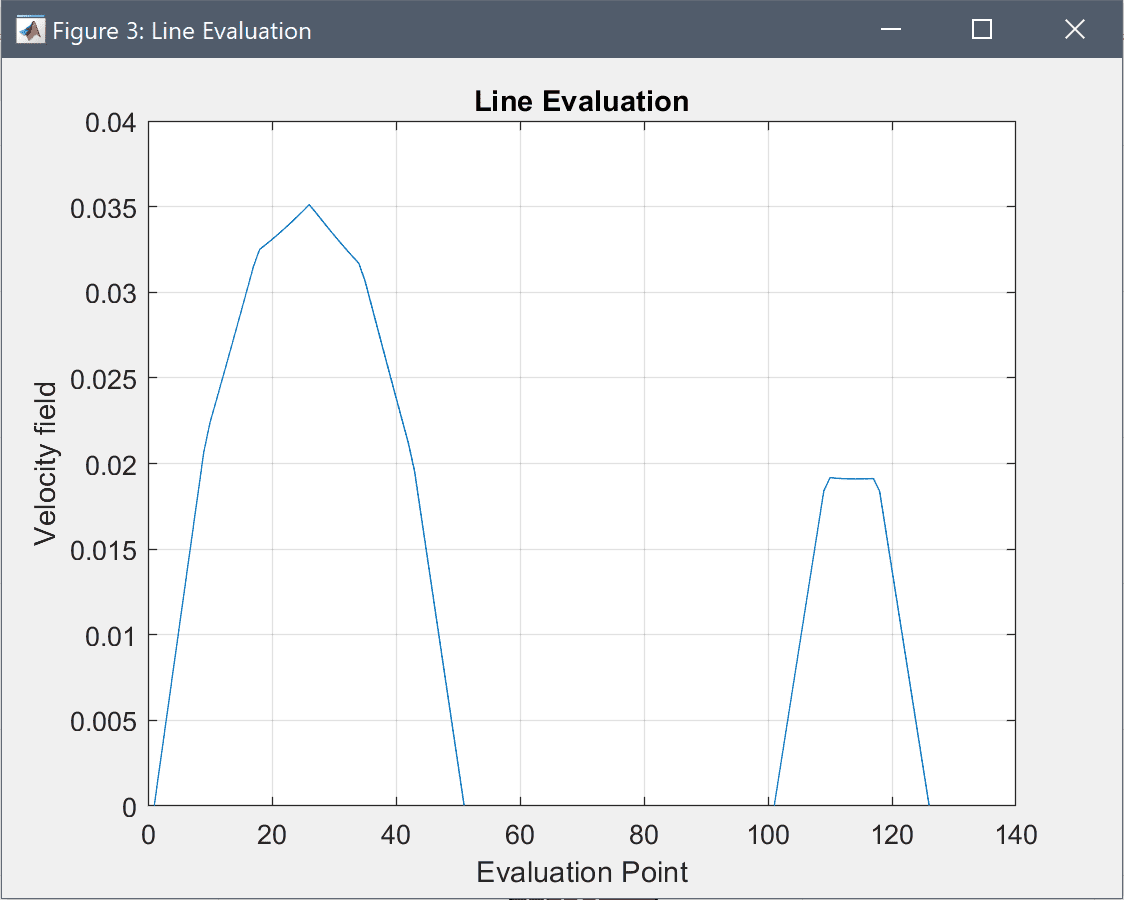The non-newtonian flow in an extrusion die fluid dynamics model has now been completed and can be saved as a binary (.fea) model file, or exported as a programmable MATLAB m-script text file, or GUI script (.fes) file.

# CLI Postprocessing

To visualize the full 3D solution the model data can be exported to the MATLAB command line interface (CLI) console with the Export Model Data Struct to MATLAB option from the File menu. The postrevolve and postplot functions can then be applied to revolve and visualize the data, for example

fea_revolved = postrevolve( fea, 24, 0.75 );

postplot( fea_revolved, 'surfexpr', 'sqrt(u^2+w^2)', ...
'parent', figure, 'axis', 'off', 'colorbar', 'off' )
view(3)
camorbit( 90, 0, 'data', 'y' )